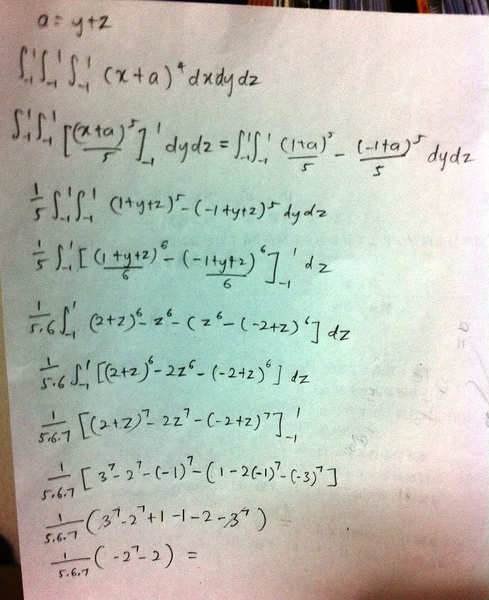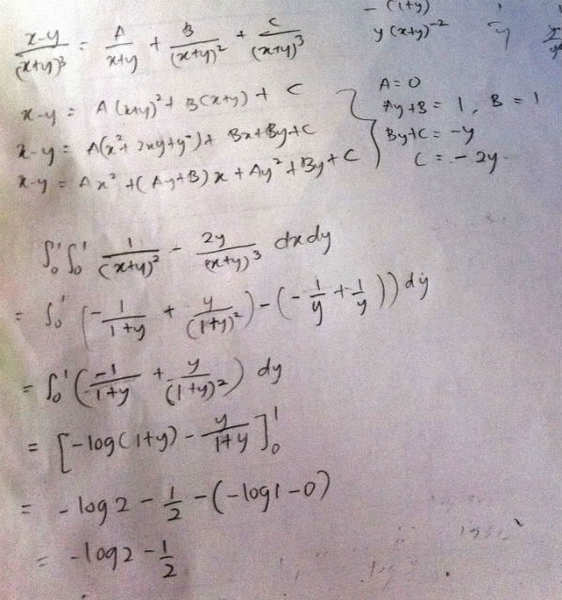# Is there a simpler way to integrate this?

Hi, I was wondering if there's a simpler way to integrate this? I got the answer by expanding one by one but that's such a long process! I got the answer 104/5 which is correct,though.

∫∫∫D (x + y + z)^4 dxdydz
D = {(x,y,z) | -1≤ x ≤1, -1≤y≤1, -1≤z≤ }

chiro
Hey aruwin.

For your integral, you are dealing with a simple rectangular volume, so the integral in its form is best evaluated by doing each variable separately at a time.

If you did it this way, then there's no point stressing about a better way to do it if you know and understand how to evaluate the integral.

you could keep in your head that the integral if (x+a)^n = (1/n)(x+a)^(n+1) if you wanted but there's nothing wrong with expanding everything out

Hey aruwin.

For your integral, you are dealing with a simple rectangular volume, so the integral in its form is best evaluated by doing each variable separately at a time.

If you did it this way, then there's no point stressing about a better way to do it if you know and understand how to evaluate the integral.

How do I do each variable sperately at a time? You mean like ∫dx∫dy∫dz ?

Ok,what about the function inside it? When say rectangle, it means
(x+y+z)2(x+y+z)2,right? But still,how do I integrate it like this?

chiro
How do I do each variable sperately at a time? You mean like ∫dx∫dy∫dz ?

Ok,what about the function inside it? When say rectangle, it means
(x+y+z)2(x+y+z)2,right? But still,how do I integrate it like this?

You do it in the form of (x+a)^n with respect to dx and integrate out the x value. Then do the same for the y and the same for the z until you get a numerical quantity.

Since x,y,z are all orthogonal variables, you can do this with no problem just like you would integrated say xydydx by keeping one variable 'constant' and then integrating it with respect to the other.

You do it in the form of (x+a)^n with respect to dx and integrate out the x value. Then do the same for the y and the same for the z until you get a numerical quantity.

Since x,y,z are all orthogonal variables, you can do this with no problem just like you would integrated say xydydx by keeping one variable 'constant' and then integrating it with respect to the other.

OK,I am gonna ask you something first, is this correct?

∫∫ (x+y+z)5/5 dydz

But what happens to the x inside the bracket?Shouldn't I do something about it?

OK,I am gonna ask you something first, is this correct?

∫∫ (x+y+z)5/5 dydz

But what happens to the x inside the bracket?Shouldn't I do something about it?

take $\int \int \int (x+y+z)^4 dx dy dz$ and stick some brackets in there $\int \int ( \int (x+y+z)^4 dx ) dy dz$
Substitute y+z = a
perform integration
substitute a = y + z
remove brackets
repeattake $\int \int \int (x+y+z)^4 dx dy dz$ and stick some brackets in there $\int \int ( \int (x+y+z)^4 dx ) dy dz$
Substitute y+z = a
perform integration
substitute a = y + z
remove brackets
repeatWon't that change the range for dx?

Won't that change the range for dx?

nope, all you've done is substitute a for y + z as to avoid confusion, you've not touched x or dx at all (you haven't really altered anything)

chiro
Won't that change the range for dx?

It only changes the interval if the interval depends on those other variables.

To understand this, you need to understand orthogonality: things are orthogonal if changing one variable doesn't change another. We can write this in terms of dA/dB = 0 and dB/dA = 0 where neither change in accordance with the other variable.

If your limits were say from 0 to y^2 - z, then yes the limit would have to factor this in but because each variable is truly orthogonal, then this doesn't happen (in this case).

take $\int \int \int (x+y+z)^4 dx dy dz$ and stick some brackets in there $\int \int ( \int (x+y+z)^4 dx ) dy dz$
Substitute y+z = a
perform integration
substitute a = y + z
remove brackets
repeatSo I did what you told me but I didn't get the answer :( Tell me what is wrong here.chiro

Even so, I won't get the answer that is 104/5.

chiro
Even so, I won't get the answer that is 104/5.

You made a major sign error in your integral:instead of + (-2 + z)^6 you wrote - (-2 + z)^6.

You made a major sign error in your integral:instead of + (-2 + z)^6 you wrote - (-2 + z)^6.

I will try again.But other than that,I'm doing this the right method,arent I?

chiro
I will try again.But other than that,I'm doing this the right method,arent I?

Yes. I checked the answer with your calculation using a calculator: it gave 20.8 which is 104/5.

Yes. I checked the answer with your calculation using a calculator: it gave 20.8 which is 104/5.

I calculated another 2 times but I didn't get the answer :( Are you sure my working there had only one mistake?

chiro
I calculated another 2 times but I didn't get the answer :( Are you sure my working there had only one mistake?

If you fix up changing the - (-2 + z) to + (-2 + z) and fix up your mistake with the 2^7 and make it 2*(-1)^7 and plug everything in correctly you will get 104/5. Show us your working so we can see what you did.

If you fix up changing the - (-2 + z) to + (-2 + z) and fix up your mistake with the 2^7 and make it 2*(-1)^7 and plug everything in correctly you will get 104/5. Show us your working so we can see what you did.

Oh,thank you!!!!I got it,I got it!!!:tongue:
By the way, I have another question on multiple integration but must I create another thread or can I just continue in here?

chiro
Oh,thank you!!!!I got it,I got it!!!:tongue:
By the way, I have another question on multiple integration but must I create another thread or can I just continue in here?

Just post it in here, it's on topic and a simple continuation of the original threads premise.

Just post it in here, it's on topic and a simple continuation of the original threads premise.

This seems a little more complicated because there's fraction which is hard to decompose and I don't know how to decompose it anyway. Can you help me out with this one?

J = ∫∫D [(x-y)/(x+y)^3] dxdy

D = {(x,y) | 0 ≤x≤1, 0≤y≤1}

Mute
Homework Helper
This seems a little more complicated because there's fraction which is hard to decompose and I don't know how to decompose it anyway. Can you help me out with this one?

J = ∫∫D [(x-y)/(x+y)^3] dxdy

D = {(x,y) | 0 ≤x≤1, 0≤y≤1}

Try the substitution u = x - y, v = x+y. Remember to work out the Jacobian and the new limits.

Try the substitution u = x - y, v = x+y. Remember to work out the Jacobian and the new limits.

I did it the other way,though. But I didn't get the answer that I am supposed to. Can you check for me,please?Mute
Homework Helper
The integral of y/(1+y)2 is not just -y/(1+y). You missed a term.

By the way, this is actually a pretty insidious integral. After you add the term you missed and get the result, try doing the integral in the opposite order (y first, then x). My earlier suggestion to try a substitution might not be a good alternate way to do this in light of this result.

Last edited:
The integral of y/(1+y)2 is not just -y/(1+y). You missed a term.

By the way, this is actually a pretty insidious integral. After you add the term you missed and get the result, try doing the integral in the opposite order (y first, then x). My earlier suggestion to try a substitution might not be a good alternate way to do this in light of this result.

Yes, I got it! The integral that I got is -1/2 but how come the answer that my professor gave is ∞? Is there any explanation to that?